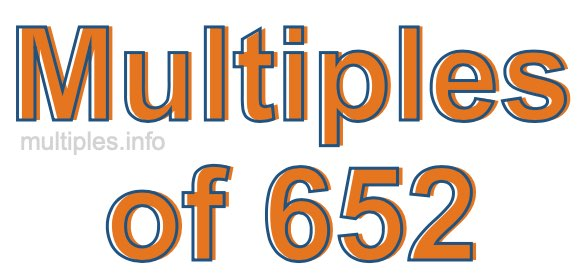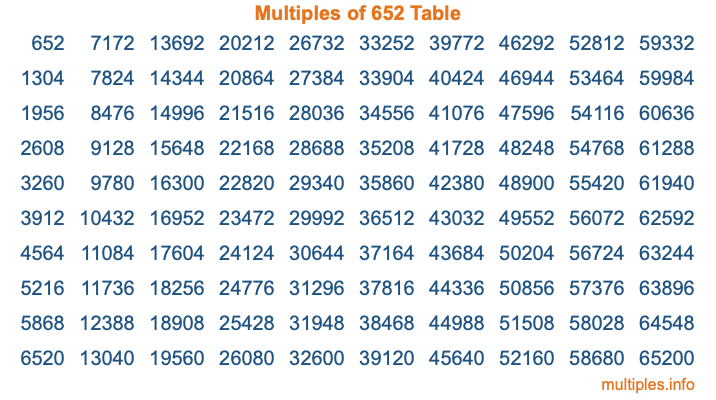Multiples of 652Welcome to the Multiples of 652 page. Here we will first teach you everything you will ever need to know about the multiples of 652, and then give you a study guide summary of everything we taught you to make sure you remember it all. Use this page to look up facts and learn information about the multiples of 652. This page will make you a multiples of six hundred fifty-two expert!

Definition of Multiples of 652
Multiples of 652 are all the numbers that when divided by 652 equal an integer. Each of the multiples of 652 are called a multiple. A multiple of 652 is created by multiplying 652 by an integer.

Therefore, to create a list of multiples of 652, you start with 1 multiplied by 652, then 2 multiplied by 652, then 3 multiplied by 652, and so on for as long as you want. Thus, the list of the first five multiples of 652 is 652, 1304, 1956, 2608, and 3260. To see a larger list of multiples of 652, see the printable image of Multiples of 652 further down on this page. We also have a category where you can choose any nth multiple of 652.

Multiples of 652 Checker
The Multiples of 652 Checker below checks to see if any number of your choice is a multiple of 652. In other words, it checks to see if there is any number (integer) that when multiplied by 652 will equal your number. To do that, we divide your number by 652. If the the quotient is an integer, then your number is a multiple of 652.

Is  a multiple of 652?

Least Common Multiple of 652 and ...
A Least Common Multiple (LCM) is the lowest multiple that two or more numbers have in common. This is also called the smallest common multiple or lowest common multiple and is useful to know when you are adding our subtracting fractions. Enter one or more numbers below (652 is already entered) to find the LCM.

Check out our LCM Calculator if you need more details about the Least Common Multiple or if you need the LCM for different numbers for adding and subtraction fractions.

nth Multiple of 652
As we stated above, 652 is the first multiple of 652, 1304 is the second multiple of 652, 1956 is the third multiple of 652, and so on. Enter a number below to find the nth multiple of 652.

th multiple of 652

Multiples of 652 vs Factors of 652
652 is a multiple of 652 and a factor of 652, but that is where the similarities end. All postive multiples of 652 are 652 or greater than 652. All positive factors of 652 are 652 or less than 652.

Below is the beginning list of multiples of 652 and the factors of 652 so you can compare:

Multiples of 652: 652, 1304, 1956, 2608, 3260, etc.

Factors of 652: 1, 2, 4, 163, 326, 652

As you can see, the multiples of 652 are all the numbers that you can divide by 652 to get a whole number. The factors of 652, on the other hand, are all the whole numbers that you can multiply by another whole number to get 652.

It's also interesting to note that if a number (x) is a factor of 652, then 652 will also be a multiple of that number (x).

Multiples of 652 vs Divisors of 652
The divisors of 652 are all the integers that 652 can be divided by evenly. Below is a list of the divisors of 652.

Divisors of 652: 1, 2, 4, 163, 326, 652

The interesting thing to note here is that if you take any multiple of 652 and divide it by a divisor of 652, you will see that the quotient is an integer.

Multiples of 652 Table
Below is an image of the first 100 multiples of 652 in a table. The table is in chronological order, column by column. The first column has the first ten multiples of 652, the second column has the next ten multiples of 652, and so on.The Multiples of 652 Table is also referred to as the 652 Times Table or Times Table of 652. You are welcome to print out our table for your studies.

Negative Multiples of 652
Although not often discussed or needed in math, it is worth mentioning that you can make a list of negative multiples of 652 by multiplying 652 by -1, then by -2, then by -3, and so on, to get the following list of negative multiples of 652:

-652, -1304, -1956, -2608, -3260, etc.

Multiples of 652 Summary
Below is a summary of important Multiples of 652 facts that we have discussed on this page. To retain the knowledge on this page, we recommend that you read through the summary and explain to yourself or a study partner why they hold true.

There are an infinite number of multiples of 652.

A multiple of 652 divided by 652 will equal a whole number.

652 divided by a factor of 652 equals a divisor of 652.

The nth multiple of 652 is n times 652.

The largest factor of 652 is equal to the first positive multiple of 652.

652 is a multiple of every factor of 652.

652 is a multiple of 652.

A multiple of 652 divided by a divisor of 652 equals an integer.

652 divided by a divisor of 652 equals a factor of 652.

Any integer times 652 will equal a multiple of 652.

Multiples of a Number
Here you can get the multiples of another number, all with the same attention to detail as we did for multiples of 652 on this page.

Multiples of
Multiples of 653
Did you find our page about multiples of six hundred fifty-two educational? Do you want more knowledge? Check out the multiples of the next number on our list!

Copyright  |   Privacy Policy  |   Disclaimer  |   Contact